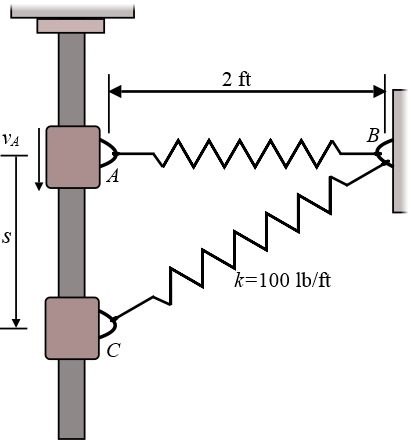# The 3 lb block is given an initial velocity of 26 ft/s when it is at A. If the spring has an...

## Question:

The 3 lb block is given an initial velocity of 26 ft/s when it is at A. If the spring has an unstretched length of 2 ft and a stiffness of k = 100 lb/ft determine the velocity of the block when s = 1 ft.## Spring Force

Spring force is the force exerted by spring when it is either stretched or compressed, against the direction of motion. It is called restoring force, as when the external force is removed it returns back to its original position.

## Answer and Explanation: 1

Become a Study.com member to unlock this answer! Create your account

List down the given data.

• The weight of block is {eq}{W_B} = 3\;{\rm{lb}} {/eq}.
• The velocity of block is {eq}{v_A} = 26{{{\rm{f}}t}...

See full answer below.

#### Learn more about this topic:Practice Applying Spring Constant Formulas

from

Chapter 17 / Lesson 11
3.4K

In this lesson, you'll have the chance to practice using the spring constant formula. The lesson includes four problems of medium difficulty involving a variety of real-life applications.